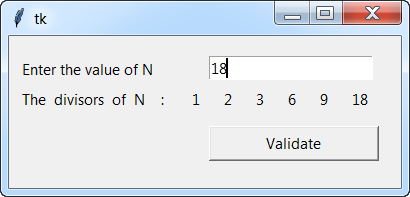## Tuesday, August 25, 2020

Solution Exercise 201: Get the divisors of an integer N graphically in python tkinter

#### Exercise 201

1. Write a program in Python that lists all divisors of a given integer N.
2. Create a program in Python that which a Tkinter window asking the user to enter an integer N and returns all the divisors of N.#### Solution

1. List of divisors of an integer N

``````def listDivisors (N):
# initialization of the list of divisors  L = []
L = []
# We browse throught the integers: 1 2 3 ... N
for i in range (1, N + 1):
# we test if the integer i is a divisor of N and we add it to the list of divisors L
if N% i == 0:
L. append (i)
return L
# We test the algorithm
print ("The list of divisors of 18 is :", listDivisors (18))
# Displays: The list of divisors of 18 is : [1, 2, 3, 6, 9, 18]
``````

2. List of divisors graphically in python tkinter
``````from tkinter import *

# method that performs the action
def action ():

# retrieve the value of the edit control
N = int (entryNumber1.get ())
lblDivisors ['text'] = 'The  divisors  of  N    :    '

# find the divisors s of n by going through all the integers from 1 to n
for i in range (1, N + 1):
if ( N%i == 0 ):
lblDivisors ['text'] = lblDivisors ['text'] + "   " + str(i) + "   "

# Creation of the main window
fen = Tk ()
fen.geometry ("400x175")

# input field for the integer N with the associated label
lblnumber1 = Label (fen, text = "Enter the value of N")
lblnumber1.place (x = 10, y = 20)
entryNumber1 = Entry (fen)
entryNumber1.place (x = 200, y = 20)

# Label which gets the result
lblDivisors = Label (fen, text = "The divisors of N : ")
lblDivisors.place (x = 10, y = 50)

# validation button
Validate = Button (fen, text = "Validate", width = 20, command = action)
Validate.place (x = 200, y = 90)

fen.mainloop ()
``````

Younes Derfoufi
my-courses.net

## Python Exercises With Solutions

1. Python Arithmetic Exercises
2. Equations & System Of Equations
3. Polynomials and Functions
4. Python Arithmetic Exercises
5. Numpy, Matplolib, Sympy , Scipy - Exercises
6. Python Arithmetic Exercises

Younes Derfoufi

## Sample Text